# Automata Theory Introduction

## Automata – What is it?

The term "Automata" is derived from the Greek word "αὐτόματα" which means "self-acting". An automaton (Automata in plural) is an abstract self-propelled computing device which follows a predetermined sequence of operations automatically.

An automaton with a finite number of states is called a Finite Automaton (FA) or Finite State Machine (FSM).

### Formal definition of a Finite Automaton

An automaton can be represented by a 5-tuple (Q, ∑, δ, q0, F), where −

• Q is a finite set of states.

• is a finite set of symbols, called the alphabet of the automaton.

• δ is the transition function.

• q0 is the initial state from where any input is processed (q0 ∈ Q).

• F is a set of final state/states of Q (F ⊆ Q).

## Related Terminologies

### Alphabet

• Definition − An alphabet is any finite set of symbols.

• Example − ∑ = {a, b, c, d} is an alphabet set where ‘a’, ‘b’, ‘c’, and ‘d’ are symbols.

### String

• Definition − A string is a finite sequence of symbols taken from ∑.

• Example − ‘cabcad’ is a valid string on the alphabet set ∑ = {a, b, c, d}

### Length of a String

• Definition − It is the number of symbols present in a string. (Denoted by |S|).

• Examples

• If S = ‘cabcad’, |S|= 6

• If |S|= 0, it is called an empty string (Denoted by λ or ε)

### Kleene Star

• Definition − The Kleene star, ∑*, is a unary operator on a set of symbols or strings, , that gives the infinite set of all possible strings of all possible lengths over including λ.

• Representation − ∑* = ∑0 ∪ ∑1 ∪ ∑2 ∪……. where ∑p is the set of all possible strings of length p.

• Example − If ∑ = {a, b}, ∑* = {λ, a, b, aa, ab, ba, bb,………..}

### Kleene Closure / Plus

• Definition − The set + is the infinite set of all possible strings of all possible lengths over ∑ excluding λ.

• Representation − ∑+ = ∑1 ∪ ∑2 ∪ ∑3 ∪…….

+ = ∑* − { λ }

• Example − If ∑ = { a, b } , ∑+ = { a, b, aa, ab, ba, bb,………..}

### Language

• Definition − A language is a subset of ∑* for some alphabet ∑. It can be finite or infinite.

• Example − If the language takes all possible strings of length 2 over ∑ = {a, b}, then L = { ab, aa, ba, bb }

# Deterministic Finite Automaton

Finite Automaton can be classified into two types −

• Deterministic Finite Automaton (DFA)
• Non-deterministic Finite Automaton (NDFA / NFA)

## Deterministic Finite Automaton (DFA)

In DFA, for each input symbol, one can determine the state to which the machine will move. Hence, it is called Deterministic Automaton. As it has a finite number of states, the machine is called Deterministic Finite Machine or Deterministic Finite Automaton.

## Formal Definition of a DFA

A DFA can be represented by a 5-tuple (Q, ∑, δ, q0, F) where −

• Q is a finite set of states.

• is a finite set of symbols called the alphabet.

• δ is the transition function where δ: Q × ∑ → Q

• q0 is the initial state from where any input is processed (q0 ∈ Q).

• F is a set of final state/states of Q (F ⊆ Q).

## Graphical Representation of a DFA

A DFA is represented by digraphs called state diagram.

• The vertices represent the states.
• The arcs labeled with an input alphabet show the transitions.
• The initial state is denoted by an empty single incoming arc.
• The final state is indicated by double circles.

### Example

Let a deterministic finite automaton be →

• Q = {a, b, c},
• ∑ = {0, 1},
• q0 = {a},
• F = {c}, and

Transition function δ as shown by the following table −

Present State Next State for Input 0 Next State for Input 1
a a b
b c a
c b c

Its graphical representation would be as follows −# Non-deterministic Finite Automaton

In NDFA, for a particular input symbol, the machine can move to any combination of the states in the machine. In other words, the exact state to which the machine moves cannot be determined. Hence, it is called Non-deterministic Automaton. As it has finite number of states, the machine is called Non-deterministic Finite Machine or Non-deterministic Finite Automaton.

### Formal Definition of an NDFA

An NDFA can be represented by a 5-tuple (Q, ∑, δ, q0, F) where −

• Q is a finite set of states.

• is a finite set of symbols called the alphabets.

• δ is the transition function where δ: Q × ∑ → 2Q

(Here the power set of Q (2Q) has been taken because in case of NDFA, from a state, transition can occur to any combination of Q states)

• q0 is the initial state from where any input is processed (q0 ∈ Q).

• F is a set of final state/states of Q (F ⊆ Q).

### Graphical Representation of an NDFA: (same as DFA)

An NDFA is represented by digraphs called state diagram.

• The vertices represent the states.
• The arcs labeled with an input alphabet show the transitions.
• The initial state is denoted by an empty single incoming arc.
• The final state is indicated by double circles.

Example

Let a non-deterministic finite automaton be →

• Q = {a, b, c}
• ∑ = {0, 1}
• q0 = {a}
• F = {c}

The transition function δ as shown below −

Present State Next State for Input 0 Next State for Input 1
a a, b b
b c a, c
c b, c c

Its graphical representation would be as follows −## DFA vs NDFA

The following table lists the differences between DFA and NDFA.

DFA NDFA
The transition from a state is to a single particular next state for each input symbol. Hence it is called deterministic. The transition from a state can be to multiple next states for each input symbol. Hence it is called non-deterministic.
Empty string transitions are not seen in DFA. NDFA permits empty string transitions.
Backtracking is allowed in DFA In NDFA, backtracking is not always possible.
Requires more space. Requires less space.
A string is accepted by a DFA, if it transits to a final state. A string is accepted by a NDFA, if at least one of all possible transitions ends in a final state.

## Acceptors, Classifiers, and Transducers

### Acceptor (Recognizer)

An automaton that computes a Boolean function is called an acceptor. All the states of an acceptor is either accepting or rejecting the inputs given to it.

### Classifier

A classifier has more than two final states and it gives a single output when it terminates.

### Transducer

An automaton that produces outputs based on current input and/or previous state is called a transducer. Transducers can be of two types −

• Mealy Machine − The output depends both on the current state and the current input.

• Moore Machine − The output depends only on the current state.

## Acceptability by DFA and NDFA

A string is accepted by a DFA/NDFA iff the DFA/NDFA starting at the initial state ends in an accepting state (any of the final states) after reading the string wholly.

A string S is accepted by a DFA/NDFA (Q, ∑, δ, q0, F), iff

δ*(q0, S) ∈ F

The language L accepted by DFA/NDFA is

{S | S ∈ ∑* and δ*(q0, S) ∈ F}

A string S′ is not accepted by a DFA/NDFA (Q, ∑, δ, q0, F), iff

δ*(q0, S′) ∉ F

The language L′ not accepted by DFA/NDFA (Complement of accepted language L) is

{S | S ∈ ∑* and δ*(q0, S) ∉ F}

Example

Let us consider the DFA shown in Figure 1.3. From the DFA, the acceptable strings can be derived.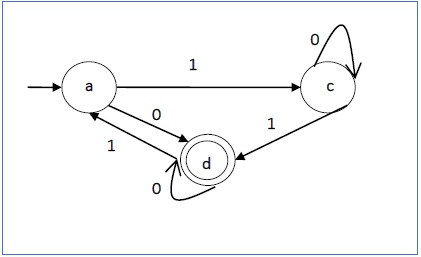Strings accepted by the above DFA: {0, 00, 11, 010, 101, ...........}

Strings not accepted by the above DFA: {1, 011, 111, ........}

# NDFA to DFA Conversion

## Problem Statement

Let X = (Qx, ∑, δx, q0, Fx) be an NDFA which accepts the language L(X). We have to design an equivalent DFA Y = (Qy, ∑, δy, q0, Fy) such that L(Y) = L(X). The following procedure converts the NDFA to its equivalent DFA −

## Algorithm

Input − An NDFA

Output − An equivalent DFA

Step 1 − Create state table from the given NDFA.

Step 2 − Create a blank state table under possible input alphabets for the equivalent DFA.

Step 3 − Mark the start state of the DFA by q0 (Same as the NDFA).

Step 4 − Find out the combination of States {Q0, Q1,... , Qn} for each possible input alphabet.

Step 5 − Each time we generate a new DFA state under the input alphabet columns, we have to apply step 4 again, otherwise go to step 6.

Step 6 − The states which contain any of the final states of the NDFA are the final states of the equivalent DFA.

## Example

Let us consider the NDFA shown in the figure below.q δ(q,0) δ(q,1)
a {a,b,c,d,e} {d,e}
b {c} {e}
c {b}
d {e}
e

Using the above algorithm, we find its equivalent DFA. The state table of the DFA is shown in below.

q δ(q,0) δ(q,1)
[a] [a,b,c,d,e] [d,e]
[a,b,c,d,e] [a,b,c,d,e] [b,d,e]
[d,e] [e]
[b,d,e] [c,e] [e]
[e]
[c, e] [b]
[b] [c] [e]
[c] [b]

The state diagram of the DFA is as follows −# DFA Minimization

## DFA Minimization using Myhill-Nerode Theorem

### Algorithm

Input − DFA

Output − Minimized DFA

Step 1 − Draw a table for all pairs of states (Qi, Qj) not necessarily connected directly [All are unmarked initially]

Step 2 − Consider every state pair (Qi, Qj) in the DFA where Qi ∈ F and Qj ∉ F or vice versa and mark them. [Here F is the set of final states]

Step 3 − Repeat this step until we cannot mark anymore states −

If there is an unmarked pair (Qi, Qj), mark it if the pair {δ (Qi, A), δ (Qi, A)} is marked for some input alphabet.

Step 4 − Combine all the unmarked pair (Qi, Qj) and make them a single state in the reduced DFA.

### Example

Let us use Algorithm 2 to minimize the DFA shown below.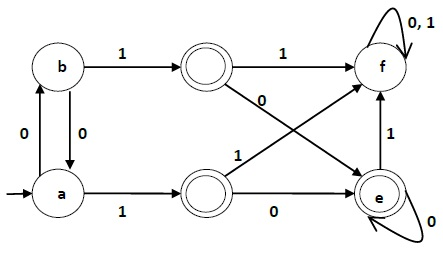Step 1 − We draw a table for all pair of states.

 a b c d e f a b c d e f

Step 2 − We mark the state pairs.

 a b c d e f a b c ✔ ✔ d ✔ ✔ e ✔ ✔ f ✔ ✔ ✔

Step 3 − We will try to mark the state pairs, with green colored check mark, transitively. If we input 1 to state ‘a’ and ‘f’, it will go to state ‘c’ and ‘f’ respectively. (c, f) is already marked, hence we will mark pair (a, f). Now, we input 1 to state ‘b’ and ‘f’; it will go to state ‘d’ and ‘f’ respectively. (d, f) is already marked, hence we will mark pair (b, f).

 a b c d e f a b c ✔ ✔ d ✔ ✔ e ✔ ✔ f ✔ ✔ ✔ ✔ ✔

After step 3, we have got state combinations {a, b} {c, d} {c, e} {d, e} that are unmarked.

We can recombine {c, d} {c, e} {d, e} into {c, d, e}

Hence we got two combined states as − {a, b} and {c, d, e}

So the final minimized DFA will contain three states {f}, {a, b} and {c, d, e}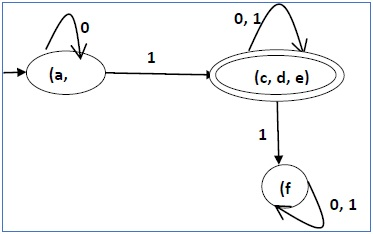## DFA Minimization using Equivalence Theorem

If X and Y are two states in a DFA, we can combine these two states into {X, Y} if they are not distinguishable. Two states are distinguishable, if there is at least one string S, such that one of δ (X, S) and δ (Y, S) is accepting and another is not accepting. Hence, a DFA is minimal if and only if all the states are distinguishable.

### Algorithm 3

Step 1 − All the states Q are divided in two partitions − final states and non-final states and are denoted by P0. All the states in a partition are 0th equivalent. Take a counter k and initialize it with 0.

Step 2 − Increment k by 1. For each partition in Pk, divide the states in Pk into two partitions if they are k-distinguishable. Two states within this partition X and Y are k-distinguishable if there is an input S such that δ(X, S) and δ(Y, S) are (k-1)-distinguishable.

Step 3 − If Pk ≠ Pk-1, repeat Step 2, otherwise go to Step 4.

Step 4 − Combine kth equivalent sets and make them the new states of the reduced DFA.

### Example

Let us consider the following DFA −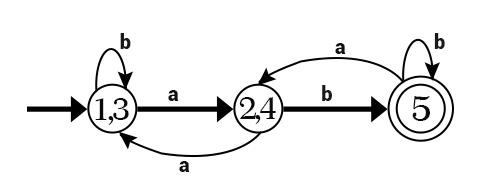q δ(q,0) δ(q,1)
a b c
b a d
c e f
d e f
e e f
f f f

Let us apply the above algorithm to the above DFA −

• P0 = {(c,d,e), (a,b,f)}
• P1 = {(c,d,e), (a,b),(f)}
• P2 = {(c,d,e), (a,b),(f)}

Hence, P1 = P2.

There are three states in the reduced DFA. The reduced DFA is as follows −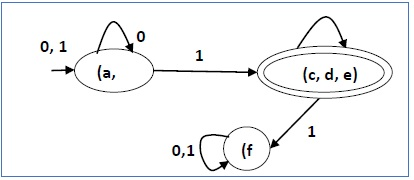Q δ(q,0) δ(q,1)
(a, b) (a, b) (c,d,e)
(c,d,e) (c,d,e) (f)
(f) (f) (f)

# Moore and Mealy Machines

Finite automata may have outputs corresponding to each transition. There are two types of finite state machines that generate output −

• Mealy Machine
• Moore machine

### Mealy Machine

A Mealy Machine is an FSM whose output depends on the present state as well as the present input.

It can be described by a 6 tuple (Q, ∑, O, δ, X, q0) where −

• Q is a finite set of states.

• is a finite set of symbols called the input alphabet.

• O is a finite set of symbols called the output alphabet.

• δ is the input transition function where δ: Q × ∑ → Q

• X is the output transition function where X: Q × ∑ → O

• q0 is the initial state from where any input is processed (q0 ∈ Q).

The state table of a Mealy Machine is shown below −

Present state Next state
input = 0 input = 1
State Output State Output
→ a b x1 c x1
b b x2 d x3
c d x3 c x1
d d x3 d x2

The state diagram of the above Mealy Machine is −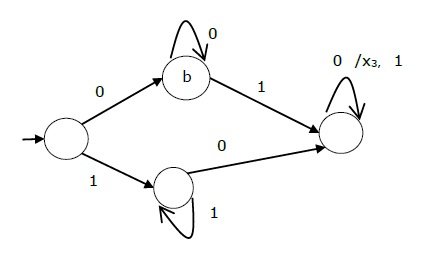### Moore Machine

Moore machine is an FSM whose outputs depend on only the present state.

A Moore machine can be described by a 6 tuple (Q, ∑, O, δ, X, q0) where −

• Q is a finite set of states.

• is a finite set of symbols called the input alphabet.

• O is a finite set of symbols called the output alphabet.

• δ is the input transition function where δ: Q × ∑ → Q

• X is the output transition function where X: Q → O

• q0 is the initial state from where any input is processed (q0 ∈ Q).

The state table of a Moore Machine is shown below −

Present state Next State Output
Input = 0 Input = 1
→ a b c x2
b b d x1
c c d x2
d d d x3

The state diagram of the above Moore Machine is −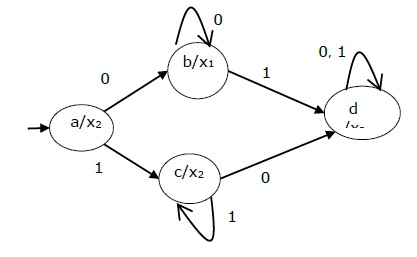### Mealy Machine vs. Moore Machine

The following table highlights the points that differentiate a Mealy Machine from a Moore Machine.

Mealy Machine Moore Machine
Output depends both upon the present state and the present input Output depends only upon the present state.
Generally, it has fewer states than Moore Machine. Generally, it has more states than Mealy Machine.
The value of the output function is a function of the transitions and the changes, when the input logic on the present state is done. The value of the output function is a function of the current state and the changes at the clock edges, whenever state changes occur.
Mealy machines react faster to inputs. They generally react in the same clock cycle. In Moore machines, more logic is required to decode the outputs resulting in more circuit delays. They generally react one clock cycle later.

## Moore Machine to Mealy Machine

### Algorithm 4

Input − Moore Machine

Output − Mealy Machine

Step 1 − Take a blank Mealy Machine transition table format.

Step 2 − Copy all the Moore Machine transition states into this table format.

Step 3 − Check the present states and their corresponding outputs in the Moore Machine state table; if for a state Qi output is m, copy it into the output columns of the Mealy Machine state table wherever Qi appears in the next state.

### Example

Let us consider the following Moore machine −

Present State Next State Output
a = 0 a = 1
→ a d b 1
b a d 0
c c c 0
d b a 1

Now we apply Algorithm 4 to convert it to Mealy Machine.

Step 1 & 2

Present State Next State
a = 0 a = 1
State Output State Output
→ a d b
b a d
c c c
d b a

Step 3

Present State Next State
a = 0 a = 1
State Output State Output
=> a d 1 b 0
b a 1 d 1
c c 0 c 0
d b 0 a 1

## Mealy Machine to Moore Machine

### Algorithm 5

Input − Mealy Machine

Output − Moore Machine

Step 1 − Calculate the number of different outputs for each state (Qi) that are available in the state table of the Mealy machine.

Step 2 − If all the outputs of Qi are same, copy state Qi. If it has n distinct outputs, break Qi into n states as Qin where n = 0, 1, 2.......

Step 3 − If the output of the initial state is 1, insert a new initial state at the beginning which gives 0 output.

### Example

Let us consider the following Mealy Machine −

Present State Next State
a = 0 a = 1
Next State Output Next State Output
→ a d 0 b 1
b a 1 d 0
c c 1 c 0
d b 0 a 1

Here, states ‘a’ and ‘d’ give only 1 and 0 outputs respectively, so we retain states ‘a’ and ‘d’. But states ‘b’ and ‘c’ produce different outputs (1 and 0). So, we divide b into b0, b1 and c into c0, c1.

Present State Next State Output
a = 0 a = 1
→ a d b1 1
b0 a d 0
b1 a d 1
c0 c1 C0 0
c1 c1 C0 1
d b0 a 0

# Introduction to Grammars

n the literary sense of the term, grammars denote syntactical rules for conversation in natural languages. Linguistics have attempted to define grammars since the inception of natural languages like English, Sanskrit, Mandarin, etc.

The theory of formal languages finds its applicability extensively in the fields of Computer Science. Noam Chomsky gave a mathematical model of grammar in 1956 which is effective for writing computer languages.

## Grammar

A grammar G can be formally written as a 4-tuple (N, T, S, P) where −

• N or VN is a set of variables or non-terminal symbols.

• T or is a set of Terminal symbols.

• S is a special variable called the Start symbol, S ∈ N

• P is Production rules for Terminals and Non-terminals. A production rule has the form α → β, where α and β are strings on VN ∪ ∑ and least one symbol of α belongs to VN.

### Example

Grammar G1 −

({S, A, B}, {a, b}, S, {S → AB, A → a, B → b})

Here,

• S, A, and B are Non-terminal symbols;

• a and b are Terminal symbols

• S is the Start symbol, S ∈ N

• Productions, P : S → AB, A → a, B → b

### Example

Grammar G2 −

(({S, A}, {a, b}, S,{S → aAb, aA → aaAb, A → ε } )

Here,

• S and A are Non-terminal symbols.

• a and b are Terminal symbols.

• ε is an empty string.

• S is the Start symbol, S ∈ N

• Production P : S → aAb, aA → aaAb, A → ε

## Derivations from a Grammar

Strings may be derived from other strings using the productions in a grammar. If a grammar G has a production α → β, we can say that x α y derives x β y in G. This derivation is written as −

x α y G x β y

### Example

Let us consider the grammar −

G2 = ({S, A}, {a, b}, S, {S → aAb, aA → aaAb, A → ε } )

Some of the strings that can be derived are −

S ⇒ aAb using production S → aAb

⇒ aaAbb using production aA → aAb

⇒ aaaAbbb using production aA → aAb

⇒ aaabbb using production A → ε

# Language Generated by a Grammar

The set of all strings that can be derived from a grammar is said to be the language generated from that grammar. A language generated by a grammar G is a subset formally defined by

L(G)={W|W ∈ ∑*, S G W}

If L(G1) = L(G2), the Grammar G1 is equivalent to the Grammar G2.

### Example

If there is a grammar

G: N = {S, A, B} T = {a, b} P = {S → AB, A → a, B → b}

Here S produces AB, and we can replace A by a, and B by b. Here, the only accepted string is ab, i.e.,

L(G) = {ab}

### Example

Suppose we have the following grammar −

G: N = {S, A, B} T = {a, b} P = {S → AB, A → aA|a, B → bB|b}

The language generated by this grammar −

L(G) = {ab, a2b, ab2, a2b2, ………}

= {am bn | m ≥ 1 and n ≥ 1}

## Construction of a Grammar Generating a Language

We’ll consider some languages and convert it into a grammar G which produces those languages.

### Example

Problem − Suppose, L (G) = {am bn | m ≥ 0 and n > 0}. We have to find out the grammar G which produces L(G).

Solution

Since L(G) = {am bn | m ≥ 0 and n > 0}

the set of strings accepted can be rewritten as −

L(G) = {b, ab,bb, aab, abb, …….}

Here, the start symbol has to take at least one ‘b’ preceded by any number of ‘a’ including null.

To accept the string set {b, ab, bb, aab, abb, …….}, we have taken the productions −

S → aS , S → B, B → b and B → bB

S → B → b (Accepted)

S → B → bB → bb (Accepted)

S → aS → aB → ab (Accepted)

S → aS → aaS → aaB → aab(Accepted)

S → aS → aB → abB → abb (Accepted)

Thus, we can prove every single string in L(G) is accepted by the language generated by the production set.

Hence the grammar −

G: ({S, A, B}, {a, b}, S, { S → aS | B , B → b | bB })

### Example

Problem − Suppose, L (G) = {am bn | m > 0 and n ≥ 0}. We have to find out the grammar G which produces L(G).

Solution

Since L(G) = {am bn | m > 0 and n ≥ 0}, the set of strings accepted can be rewritten as −

L(G) = {a, aa, ab, aaa, aab ,abb, …….}

Here, the start symbol has to take at least one ‘a’ followed by any number of ‘b’ including null.

To accept the string set {a, aa, ab, aaa, aab, abb, …….}, we have taken the productions −

S → aA, A → aA , A → B, B → bB ,B → λ

S → aA → aB → aλ → a (Accepted)

S → aA → aaA → aaB → aaλ → aa (Accepted)

S → aA → aB → abB → abλ → ab (Accepted)

S → aA → aaA → aaaA → aaaB → aaaλ → aaa (Accepted)

S → aA → aaA → aaB → aabB → aabλ → aab (Accepted)

S → aA → aB → abB → abbB → abbλ → abb (Accepted)

Thus, we can prove every single string in L(G) is accepted by the language generated by the production set.

Hence the grammar −

G: ({S, A, B}, {a, b}, S, {S → aA, A → aA | B, B → λ | bB })

# Chomsky Classification of Grammars

According to Noam Chomosky, there are four types of grammars − Type 0, Type 1, Type 2, and Type 3. The following table shows how they differ from each other −

Grammar Type Grammar Accepted Language Accepted Automaton

Type 0 Unrestricted grammar Recursively enumerable language Turing Machine
Type 1 Context-sensitive grammar Context-sensitive language Linear-bounded automaton
Type 2 Context-free grammar Context-free language Pushdown automaton
Type 3 Regular grammar Regular language Finite state automaton

Take a look at the following illustration. It shows the scope of each type of grammar −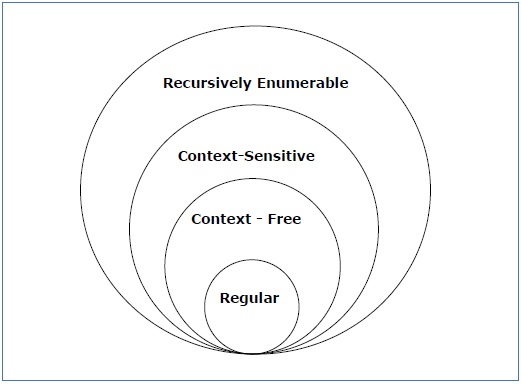## Type - 3 Grammar

Type-3 grammars generate regular languages. Type-3 grammars must have a single non-terminal on the left-hand side and a right-hand side consisting of a single terminal or single terminal followed by a single non-terminal.

The productions must be in the form X → a or X → aY

where X, Y ∈ N (Non terminal)

and a ∈ T (Terminal)

The rule S → ε is allowed if S does not appear on the right side of any rule.

### Example

```X → ε
X → a | aY
Y → b
```

## Type - 2 Grammar

Type-2 grammars generate context-free languages.

The productions must be in the form A → γ

where A ∈ N (Non terminal)

and γ ∈ (T ∪ N)* (String of terminals and non-terminals).

These languages generated by these grammars are be recognized by a non-deterministic pushdown automaton.

### Example

```S → X a
X → a
X → aX
X → abc
X → ε
```

## Type - 1 Grammar

Type-1 grammars generate context-sensitive languages. The productions must be in the form

α A β → α γ β

where A ∈ N (Non-terminal)

and α, β, γ ∈ (T ∪ N)* (Strings of terminals and non-terminals)

The strings α and β may be empty, but γ must be non-empty.

The rule S → ε is allowed if S does not appear on the right side of any rule. The languages generated by these grammars are recognized by a linear bounded automaton.

### Example

```AB → AbBc
A → bcA
B → b
```

## Type - 0 Grammar

Type-0 grammars generate recursively enumerable languages. The productions have no restrictions. They are any phase structure grammar including all formal grammars.

They generate the languages that are recognized by a Turing machine.

The productions can be in the form of α → β where α is a string of terminals and nonterminals with at least one non-terminal and α cannot be null. β is a string of terminals and non-terminals.

### Example

```S → ACaB
Bc → acB
CB → DB
```

# Regular Expressions

A Regular Expression can be recursively defined as follows −

• ε is a Regular Expression indicates the language containing an empty string. (L (ε) = {ε})

• φ is a Regular Expression denoting an empty language. (L (φ) = { })

• x is a Regular Expression where L = {x}

• If X is a Regular Expression denoting the language L(X) and Y is a Regular Expression denoting the language L(Y), then

• X + Y is a Regular Expression corresponding to the language L(X) ∪ L(Y) where L(X+Y) = L(X) ∪ L(Y).

• X . Y is a Regular Expression corresponding to the language L(X) . L(Y) where L(X.Y) = L(X) . L(Y)

• R* is a Regular Expression corresponding to the language L(R*)where L(R*) = (L(R))*

• If we apply any of the rules several times from 1 to 5, they are Regular Expressions.

## Some RE Examples

Regular Expressions Regular Set
(0 + 10*) L = { 0, 1, 10, 100, 1000, 10000, … }
(0*10*) L = {1, 01, 10, 010, 0010, …}
(0 + ε)(1 + ε) L = {ε, 0, 1, 01}
(a+b)* Set of strings of a’s and b’s of any length including the null string. So L = { ε, a, b, aa , ab , bb , ba, aaa…….}
(a+b)*abb Set of strings of a’s and b’s ending with the string abb. So L = {abb, aabb, babb, aaabb, ababb, …………..}
(11)* Set consisting of even number of 1’s including empty string, So L= {ε, 11, 1111, 111111, ……….}
(aa)*(bb)*b Set of strings consisting of even number of a’s followed by odd number of b’s , so L = {b, aab, aabbb, aabbbbb, aaaab, aaaabbb, …………..}
(aa + ab + ba + bb)* String of a’s and b’s of even length can be obtained by concatenating any combination of the strings aa, ab, ba and bb including null, so L = {aa, ab, ba, bb, aaab, aaba, …………..}

# Regular Sets

Any set that represents the value of the Regular Expression is called a Regular Set.

### Properties of Regular Sets

Property 1. The union of two regular set is regular.

Proof

Let us take two regular expressions

RE1 = a(aa)* and RE2 = (aa)*

So, L1 = {a, aaa, aaaaa,.....} (Strings of odd length excluding Null)

and L2 ={ ε, aa, aaaa, aaaaaa,.......} (Strings of even length including Null)

L1 ∪ L2 = { ε, a, aa, aaa, aaaa, aaaaa, aaaaaa,.......}

(Strings of all possible lengths including Null)

RE (L1 ∪ L2) = a* (which is a regular expression itself)

Hence, proved.

Property 2. The intersection of two regular set is regular.

Proof

Let us take two regular expressions

RE1 = a(a*) and RE2 = (aa)*

So, L1 = { a,aa, aaa, aaaa, ....} (Strings of all possible lengths excluding Null)

L2 = { ε, aa, aaaa, aaaaaa,.......} (Strings of even length including Null)

L1 ∩ L2 = { aa, aaaa, aaaaaa,.......} (Strings of even length excluding Null)

RE (L1 ∩ L2) = aa(aa)* which is a regular expression itself.

Hence, proved.

Property 3. The complement of a regular set is regular.

Proof

Let us take a regular expression −

RE = (aa)*

So, L = {ε, aa, aaaa, aaaaaa, .......} (Strings of even length including Null)

Complement of L is all the strings that is not in L.

So, L’ = {a, aaa, aaaaa, .....} (Strings of odd length excluding Null)

RE (L’) = a(aa)* which is a regular expression itself.

Hence, proved.

Property 4. The difference of two regular set is regular.

Proof

Let us take two regular expressions −

RE1 = a (a*) and RE2 = (aa)*

So, L1 = {a, aa, aaa, aaaa, ....} (Strings of all possible lengths excluding Null)

L2 = { ε, aa, aaaa, aaaaaa,.......} (Strings of even length including Null)

L1 – L2 = {a, aaa, aaaaa, aaaaaaa, ....}

(Strings of all odd lengths excluding Null)

RE (L1 – L2) = a (aa)* which is a regular expression.

Hence, proved.

Property 5. The reversal of a regular set is regular.

Proof

We have to prove LR is also regular if L is a regular set.

Let, L = {01, 10, 11, 10}

RE (L) = 01 + 10 + 11 + 10

LR = {10, 01, 11, 01}

RE (LR) = 01 + 10 + 11 + 10 which is regular

Hence, proved.

Property 6. The closure of a regular set is regular.

Proof

If L = {a, aaa, aaaaa, .......} (Strings of odd length excluding Null)

i.e., RE (L) = a (aa)*

L* = {a, aa, aaa, aaaa , aaaaa,……………} (Strings of all lengths excluding Null)

RE (L*) = a (a)*

Hence, proved.

Property 7. The concatenation of two regular sets is regular.

Proof −

Let RE1 = (0+1)*0 and RE2 = 01(0+1)*

Here, L1 = {0, 00, 10, 000, 010, ......} (Set of strings ending in 0)

and L2 = {01, 010,011,.....} (Set of strings beginning with 01)

Then, L1 L2 = {001,0010,0011,0001,00010,00011,1001,10010,.............}

Set of strings containing 001 as a substring which can be represented by an RE − (0 + 1)*001(0 + 1)*

Hence, proved.

## Identities Related to Regular Expressions

Given R, P, L, Q as regular expressions, the following identities hold −

• ∅* = ε
• ε* = ε
• RR* = R*R
• R*R* = R*
• (R*)* = R*
• RR* = R*R
• (PQ)*P =P(QP)*
• (a+b)* = (a*b*)* = (a*+b*)* = (a+b*)* = a*(ba*)*
• R + ∅ = ∅ + R = R (The identity for union)
• R ε = ε R = R (The identity for concatenation)
• ∅ L = L ∅ = ∅ (The annihilator for concatenation)
• R + R = R (Idempotent law)
• L (M + N) = LM + LN (Left distributive law)
• (M + N) L = ML + NL (Right distributive law)
• ε + RR* = ε + R*R = R*

# Arden's Theorem

In order to find out a regular expression of a Finite Automaton, we use Arden’s Theorem along with the properties of regular expressions.

Statement

Let P and Q be two regular expressions.

If P does not contain null string, then R = Q + RP has a unique solution that is R = QP*

Proof

R = Q + (Q + RP)P [After putting the value R = Q + RP]

= Q + QP + RPP

When we put the value of R recursively again and again, we get the following equation −

R = Q + QP + QP2 + QP3…..

R = Q (ε + P + P2 + P3 + …. )

R = QP* [As P* represents (ε + P + P2 + P3 + ….) ]

Hence, proved.

## Assumptions for Applying Arden’s Theorem

• The transition diagram must not have NULL transitions
• It must have only one initial state

### Method

Step 1 − Create equations as the following form for all the states of the DFA having n states with initial state q1.

q1 = q1R11 + q2R21 + … + qnRn1 + ε

q2 = q1R12 + q2R22 + … + qnRn2

…………………………

…………………………

…………………………

…………………………

qn = q1R1n + q2R2n + … + qnRnn

Rij represents the set of labels of edges from qi to qj, if no such edge exists, then Rij = ∅

Step 2 − Solve these equations to get the equation for the final state in terms of Rij

Problem

Construct a regular expression corresponding to the automata given below −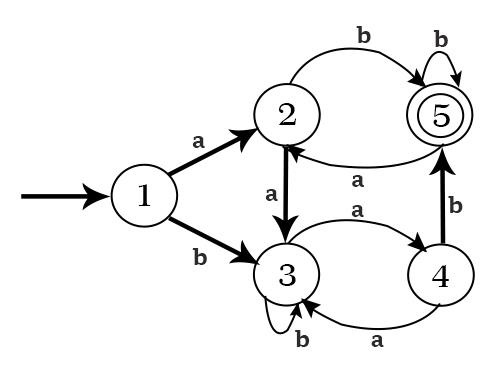Solution

Here the initial state and final state is q1.

The equations for the three states q1, q2, and q3 are as follows −

q1 = q1a + q3a + ε (ε move is because q1 is the initial state0

q2 = q1b + q2b + q3b

q3 = q2a

Now, we will solve these three equations −

q2 = q1b + q2b + q3b

= q1b + q2b + (q2a)b (Substituting value of q3)

= q1b + q2(b + ab)

= q1b (b + ab)* (Applying Arden’s Theorem)

q1 = q1a + q3a + ε

= q1a + q2aa + ε (Substituting value of q3)

= q1a + q1b(b + ab*)aa + ε (Substituting value of q2)

= q1(a + b(b + ab)*aa) + ε

= ε (a+ b(b + ab)*aa)*

= (a + b(b + ab)*aa)*

Hence, the regular expression is (a + b(b + ab)*aa)*.

Problem

Construct a regular expression corresponding to the automata given below −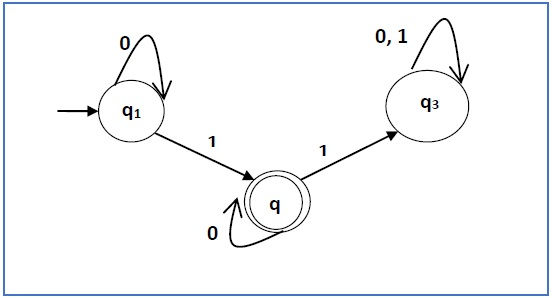Solution

Here the initial state is q1 and the final state is q2

Now we write down the equations −

q1 = q10 + ε

q2 = q11 + q20

q3 = q21 + q30 + q31

Now, we will solve these three equations −

q1 = ε0* [As, εR = R]

So, q1 = 0*

q2 = 0*1 + q20

So, q2 = 0*1(0)* [By Arden’s theorem]

Hence, the regular expression is 0*10*.

# Construction of an FA from an RE

We can use Thompson's Construction to find out a Finite Automaton from a Regular Expression. We will reduce the regular expression into smallest regular expressions and converting these to NFA and finally to DFA.

Some basic RA expressions are the following −

Case 1 − For a regular expression ‘a’, we can construct the following FA −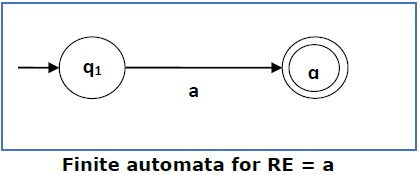Case 2 − For a regular expression ‘ab’, we can construct the following FA −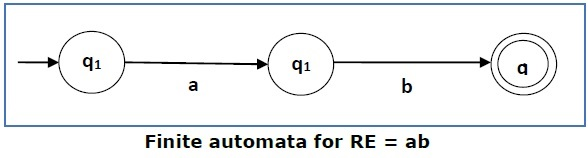Case 3 − For a regular expression (a+b), we can construct the following FA −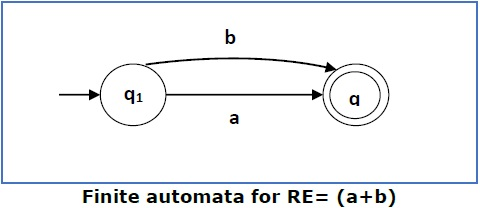Case 4 − For a regular expression (a+b)*, we can construct the following FA −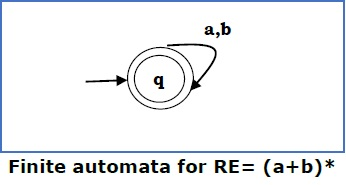### Method

Step 1 Construct an NFA with Null moves from the given regular expression.

Step 2 Remove Null transition from the NFA and convert it into its equivalent DFA.

Problem

Convert the following RA into its equivalent DFA − 1 (0 + 1)* 0

Solution

We will concatenate three expressions "1", "(0 + 1)*" and "0"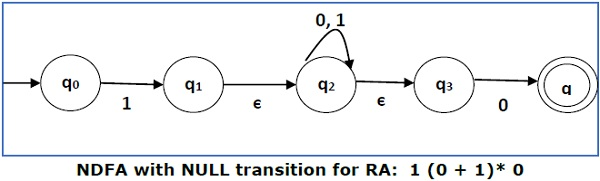Now we will remove the ε transitions. After we remove the ε transitions from the NDFA, we get the following −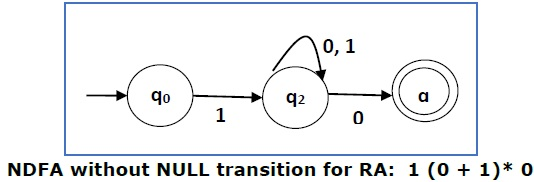It is an NDFA corresponding to the RE − 1 (0 + 1)* 0. If you want to convert it into a DFA, simply apply the method of converting NDFA to DFA discussed in Chapter 1.

## Finite Automata with Null Moves (NFA-ε)

A Finite Automaton with null moves (FA-ε) does transit not only after giving input from the alphabet set but also without any input symbol. This transition without input is called a null move.

An NFA-ε is represented formally by a 5-tuple (Q, ∑, δ, q0, F), consisting of

• Q − a finite set of states

• − a finite set of input symbols

• δ − a transition function δ : Q × (∑ ∪ {ε}) → 2Q

• q0 − an initial state q0 ∈ Q

• F − a set of final state/states of Q (F⊆Q).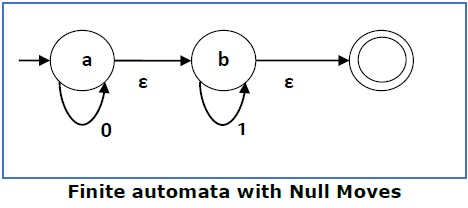The above (FA-ε) accepts a string set − {0, 1, 01}

## Removal of Null Moves from Finite Automata

If in an NDFA, there is ϵ-move between vertex X to vertex Y, we can remove it using the following steps −

• Find all the outgoing edges from Y.
• Copy all these edges starting from X without changing the edge labels.
• If X is an initial state, make Y also an initial state.
• If Y is a final state, make X also a final state.

Problem

Convert the following NFA-ε to NFA without Null move.Solution

Step 1

Here the ε transition is between q1 and q2, so let q1 is X and qf is Y.

Here the outgoing edges from qf is to qf for inputs 0 and 1.

Step 2

Now we will Copy all these edges from q1 without changing the edges from qf and get the following FA −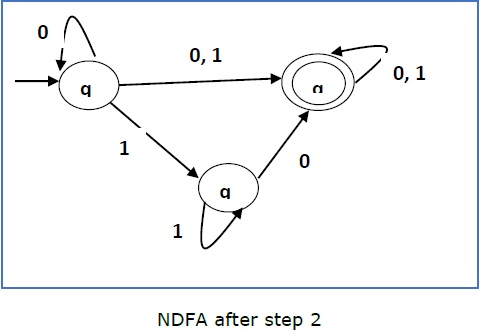Step 3

Here q1 is an initial state, so we make qf also an initial state.

So the FA becomes −Step 4

Here qf is a final state, so we make q1 also a final state.

So the FA becomes −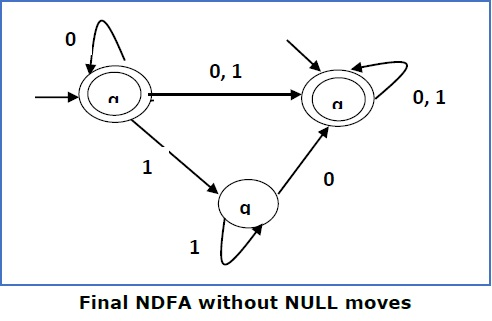# Pumping Lemma For Regular Grammars

### Theorem

Let L be a regular language. Then there exists a constant ‘c’ such that for every string w in L

|w| ≥ c

We can break w into three strings, w = xyz, such that −

• |y| > 0
• |xy| ≤ c
• For all k ≥ 0, the string xykz is also in L.

## Applications of Pumping Lemma

Pumping Lemma is to be applied to show that certain languages are not regular. It should never be used to show a language is regular.

• If L is regular, it satisfies Pumping Lemma.

• If L does not satisfy Pumping Lemma, it is non-regular.

## Method to prove that a language L is not regular

• At first, we have to assume that L is regular.

• So, the pumping lemma should hold for L.

• Use the pumping lemma to obtain a contradiction −

• Select w such that |w| ≥ c

• Select y such that |y| ≥ 1

• Select x such that |xy| ≤ c

• Assign the remaining string to z.

• Select k such that the resulting string is not in L.

Hence L is not regular.

Problem

Prove that L = {aibi | i ≥ 0} is not regular.

Solution

• At first, we assume that L is regular and n is the number of states.

• Let w = anbn. Thus |w| = 2n ≥ n.

• By pumping lemma, let w = xyz, where |xy| ≤ n.

• Let x = ap, y = aq, and z = arbn, where p + q + r = n, p ≠ 0, q ≠ 0, r ≠ 0. Thus |y| ≠ 0.

• Let k = 2. Then xy2z = apa2qarbn.

• Number of as = (p + 2q + r) = (p + q + r) + q = n + q

• Hence, xy2z = an+q bn. Since q ≠ 0, xy2z is not of the form anbn.

• Thus, xy2z is not in L. Hence L is not regular.

# DFA Complement

If (Q, ∑, δ, q0, F) be a DFA that accepts a language L, then the complement of the DFA can be obtained by swapping its accepting states with its non-accepting states and vice versa.

We will take an example and elaborate this below −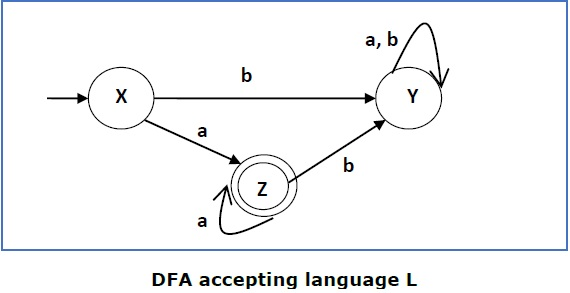This DFA accepts the language

L = {a, aa, aaa , ............. }

over the alphabet

∑ = {a, b}

So, RE = a+.

Now we will swap its accepting states with its non-accepting states and vice versa and will get the following −This DFA accepts the language

Ľ = {ε, b, ab ,bb,ba, ............... }

over the alphabet

∑ = {a, b}

Note − If we want to complement an NFA, we have to first convert it to DFA and then have to swap states as in the previous method.

# Context-Free Grammar Introduction

Definition − A context-free grammar (CFG) consisting of a finite set of grammar rules is a quadruple (N, T, P, S) where

• N is a set of non-terminal symbols.

• T is a set of terminals where N ∩ T = NULL.

• P is a set of rules, P: N → (N ∪ T)*, i.e., the left-hand side of the production rule P does have any right context or left context.

• S is the start symbol.

Example

• The grammar ({A}, {a, b, c}, P, A), P : A → aA, A → abc.
• The grammar ({S, a, b}, {a, b}, P, S), P: S → aSa, S → bSb, S → ε
• The grammar ({S, F}, {0, 1}, P, S), P: S → 00S | 11F, F → 00F | ε

## Generation of Derivation Tree

A derivation tree or parse tree is an ordered rooted tree that graphically represents the semantic information a string derived from a context-free grammar.

### Representation Technique

• Root vertex − Must be labeled by the start symbol.

• Vertex − Labeled by a non-terminal symbol.

• Leaves − Labeled by a terminal symbol or ε.

If S → x1x2 …… xn is a production rule in a CFG, then the parse tree / derivation tree will be as follows −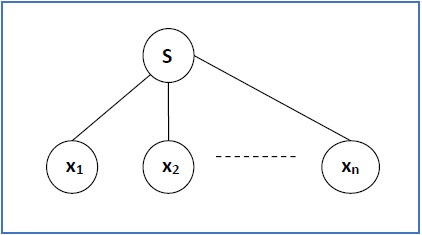There are two different approaches to draw a derivation tree −

Top-down Approach −

• Starts with the starting symbol S

• Goes down to tree leaves using productions

Bottom-up Approach −

• Starts from tree leaves

• Proceeds upward to the root which is the starting symbol S

### Derivation or Yield of a Tree

The derivation or the yield of a parse tree is the final string obtained by concatenating the labels of the leaves of the tree from left to right, ignoring the Nulls. However, if all the leaves are Null, derivation is Null.

Example

Let a CFG {N,T,P,S} be

N = {S}, T = {a, b}, Starting symbol = S, P = S → SS | aSb | ε

One derivation from the above CFG is “abaabb”

S → SS → aSbS → abS → abaSb → abaaSbb → abaabb### Sentential Form and Partial Derivation Tree

A partial derivation tree is a sub-tree of a derivation tree/parse tree such that either all of its children are in the sub-tree or none of them are in the sub-tree.

Example

If in any CFG the productions are −

S → AB, A → aaA | ε, B → Bb| ε

the partial derivation tree can be the following −If a partial derivation tree contains the root S, it is called a sentential form. The above sub-tree is also in sentential form.

### Leftmost and Rightmost Derivation of a String

• Leftmost derivation − A leftmost derivation is obtained by applying production to the leftmost variable in each step.

• Rightmost derivation − A rightmost derivation is obtained by applying production to the rightmost variable in each step.

Example

Let any set of production rules in a CFG be

X → X+X | X*X |X| a

over an alphabet {a}.

The leftmost derivation for the string "a+a*a" may be −

X → X+X → a+X → a + X*X → a+a*X → a+a*a

The stepwise derivation of the above string is shown as below −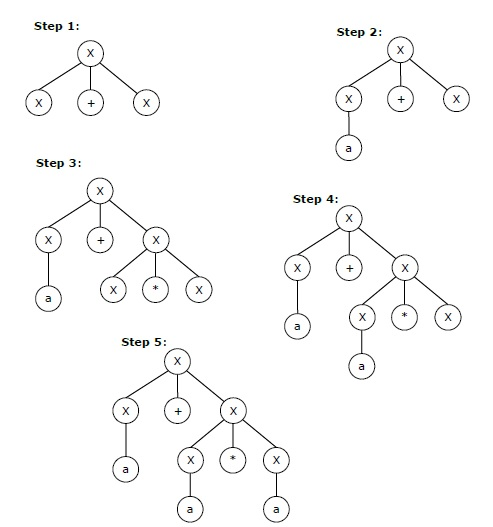The rightmost derivation for the above string "a+a*a" may be −

X → X*X → X*a → X+X*a → X+a*a → a+a*a

The stepwise derivation of the above string is shown as below −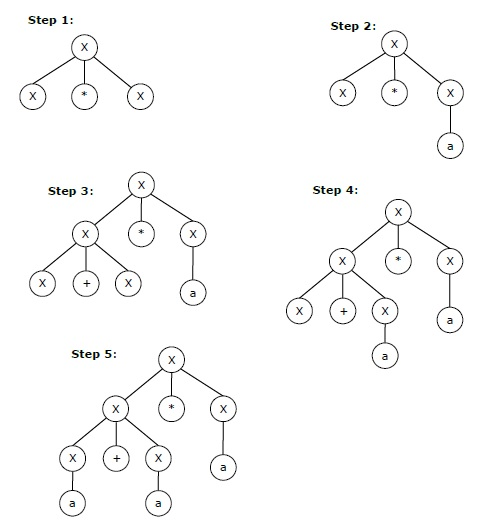## Left and Right Recursive Grammars

In a context-free grammar G, if there is a production in the form X → Xa where X is a non-terminal and ‘a’ is a string of terminals, it is called a left recursive production. The grammar having a left recursive production is called a left recursive grammar.

And if in a context-free grammar G, if there is a production is in the form X → aX where X is a non-terminal and ‘a’ is a string of terminals, it is called a right recursive production. The grammar having a right recursive production is called a right recursive grammar.

# Ambiguity in Context-Free Grammars

If a context free grammar G has more than one derivation tree for some string w ∈ L(G), it is called an ambiguous grammar. There exist multiple right-most or left-most derivations for some string generated from that grammar.

## Problem

Check whether the grammar G with production rules −

X → X+X | X*X |X| a

is ambiguous or not.

## Solution

Let’s find out the derivation tree for the string "a+a*a". It has two leftmost derivations.

Derivation 1X → X+X → a +X → a+ X*X → a+a*X → a+a*a

Parse tree 1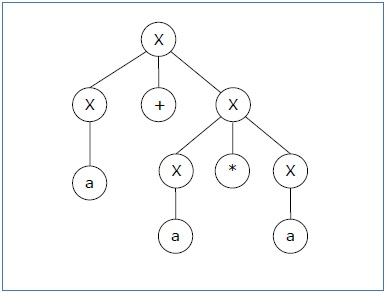Derivation 2X → X*X → X+X*X → a+ X*X → a+a*X → a+a*a

Parse tree 2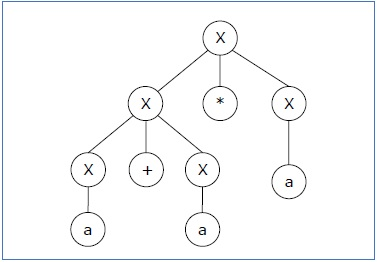Since there are two parse trees for a single string "a+a*a", the grammar G is ambiguous.

# CFL Closure Property

Context-free languages are closed under −

• Union
• Concatenation
• Kleene Star operation

## Union

Let L1 and L2 be two context free languages. Then L1 ∪ L2 is also context free.

### Example

Let L1 = { anbn , n > 0}. Corresponding grammar G1 will have P: S1 → aAb|ab

Let L2 = { cmdm , m ≥ 0}. Corresponding grammar G2 will have P: S2 → cBb| ε

Union of L1 and L2, L = L1 ∪ L2 = { anbn } ∪ { cmdm }

The corresponding grammar G will have the additional production S → S1 | S2

## Concatenation

If L1 and L2 are context free languages, then L1L2 is also context free.

### Example

Union of the languages L1 and L2, L = L1L2 = { anbncmdm }

The corresponding grammar G will have the additional production S → S1 S2

## Kleene Star

If L is a context free language, then L* is also context free.

### Example

Let L = { anbn , n ≥ 0}. Corresponding grammar G will have P: S → aAb| ε

Kleene Star L1 = { anbn }*

The corresponding grammar G1 will have additional productions S1 → SS1 | ε

Context-free languages are not closed under −

• Intersection − If L1 and L2 are context free languages, then L1 ∩ L2 is not necessarily context free.

• Intersection with Regular Language − If L1 is a regular language and L2 is a context free language, then L1 ∩ L2 is a context free language.

• Complement − If L1 is a context free language, then L1’ may not be context free.

# CFG Simplification

In a CFG, it may happen that all the production rules and symbols are not needed for the derivation of strings. Besides, there may be some null productions and unit productions. Elimination of these productions and symbols is called simplification of CFGs. Simplification essentially comprises of the following steps −

• Reduction of CFG
• Removal of Unit Productions
• Removal of Null Productions

## Reduction of CFG

CFGs are reduced in two phases −

Phase 1 − Derivation of an equivalent grammar, G’, from the CFG, G, such that each variable derives some terminal string.

Derivation Procedure

Step 1 − Include all symbols, W1, that derive some terminal and initialize i=1.

Step 2 − Include all symbols, Wi+1, that derive Wi.

Step 3 − Increment i and repeat Step 2, until Wi+1 = Wi.

Step 4 − Include all production rules that have Wi in it.

Phase 2 − Derivation of an equivalent grammar, G”, from the CFG, G’, such that each symbol appears in a sentential form.

Derivation Procedure

Step 1 − Include the start symbol in Y1 and initialize i = 1.

Step 2 − Include all symbols, Yi+1, that can be derived from Yi and include all production rules that have been applied.

Step 3 − Increment i and repeat Step 2, until Yi+1 = Yi.

### Problem

Find a reduced grammar equivalent to the grammar G, having production rules, P: S → AC | B, A → a, C → c | BC, E → aA | e

### Solution

Phase 1

T = { a, c, e }

W1 = { A, C, E } from rules A → a, C → c and E → aA

W2 = { A, C, E } U { S } from rule S → AC

W3 = { A, C, E, S } U ∅

Since W2 = W3, we can derive G’ as −

G’ = { { A, C, E, S }, { a, c, e }, P, {S}}

where P: S → AC, A → a, C → c , E → aA | e

Phase 2

Y1 = { S }

Y2 = { S, A, C } from rule S → AC

Y3 = { S, A, C, a, c } from rules A → a and C → c

Y4 = { S, A, C, a, c }

Since Y3 = Y4, we can derive G” as −

G” = { { A, C, S }, { a, c }, P, {S}}

where P: S → AC, A → a, C → c

## Removal of Unit Productions

Any production rule in the form A → B where A, B ∈ Non-terminal is called unit production..

### Removal Procedure −

Step 1 − To remove A → B, add production A → x to the grammar rule whenever B → x occurs in the grammar. [x ∈ Terminal, x can be Null]

Step 2 − Delete A → B from the grammar.

Step 3 − Repeat from step 1 until all unit productions are removed.

Problem

Remove unit production from the following −

S → XY, X → a, Y → Z | b, Z → M, M → N, N → a

Solution

There are 3 unit productions in the grammar −

Y → Z, Z → M, and M → N

At first, we will remove M → N.

As N → a, we add M → a, and M → N is removed.

The production set becomes

S → XY, X → a, Y → Z | b, Z → M, M → a, N → a

Now we will remove Z → M.

As M → a, we add Z→ a, and Z → M is removed.

The production set becomes

S → XY, X → a, Y → Z | b, Z → a, M → a, N → a

Now we will remove Y → Z.

As Z → a, we add Y→ a, and Y → Z is removed.

The production set becomes

S → XY, X → a, Y → a | b, Z → a, M → a, N → a

Now Z, M, and N are unreachable, hence we can remove those.

The final CFG is unit production free −

S → XY, X → a, Y → a | b

## Removal of Null Productions

In a CFG, a non-terminal symbol ‘A’ is a nullable variable if there is a production A → ε or there is a derivation that starts at A and finally ends up with

ε: A → .......… → ε

### Removal Procedure

Step 1 − Find out nullable non-terminal variables which derive ε.

Step 2 − For each production A → a, construct all productions A → x where x is obtained from ‘a’ by removing one or multiple non-terminals from Step 1.

Step 3 − Combine the original productions with the result of step 2 and remove ε - productions.

Problem

Remove null production from the following −

S → ASA | aB | b, A → B, B → b | ∈

Solution

There are two nullable variables − A and B

At first, we will remove B → ε.

After removing B → ε, the production set becomes −

S→ASA | aB | b | a, A ε B| b | &epsilon, B → b

Now we will remove A → ε.

After removing A → ε, the production set becomes −

S→ASA | aB | b | a | SA | AS | S, A → B| b, B → b

This is the final production set without null transition.

# Chomsky Normal Form

A CFG is in Chomsky Normal Form if the Productions are in the following forms −

• A → a
• A → BC
• S → ε

where A, B, and C are non-terminals and a is terminal.

## Algorithm to Convert into Chomsky Normal Form −

Step 1 − If the start symbol S occurs on some right side, create a new start symbol S’ and a new production S’→ S.

Step 2 − Remove Null productions. (Using the Null production removal algorithm discussed earlier)

Step 3 − Remove unit productions. (Using the Unit production removal algorithm discussed earlier)

Step 4 − Replace each production A → B1…Bn where n > 2 with A → B1C where C → B2 …Bn. Repeat this step for all productions having two or more symbols in the right side.

Step 5 − If the right side of any production is in the form A → aB where a is a terminal and A, B are non-terminal, then the production is replaced by A → XB and X → a. Repeat this step for every production which is in the form A → aB.

### Problem

Convert the following CFG into CNF

S → ASA | aB, A → B | S, B → b | ε

### Solution

(1) Since S appears in R.H.S, we add a new state S0 and S0→S is added to the production set and it becomes −

S0→S, S→ ASA | aB, A → B | S, B → b | ∈

(2) Now we will remove the null productions −

B → ∈ and A → ∈

After removing B → ε, the production set becomes −

S0→S, S→ ASA | aB | a, A → B | S | ∈, B → b

After removing A → ∈, the production set becomes −

S0→S, S→ ASA | aB | a | AS | SA | S, A → B | S, B → b

(3) Now we will remove the unit productions.

After removing S → S, the production set becomes −

S0→S, S→ ASA | aB | a | AS | SA, A → B | S, B → b

After removing S0→ S, the production set becomes −

S0→ ASA | aB | a | AS | SA, S→ ASA | aB | a | AS | SA

A → B | S, B → b

After removing A→ B, the production set becomes −

S0 → ASA | aB | a | AS | SA, S→ ASA | aB | a | AS | SA

A → S | b

B → b

After removing A→ S, the production set becomes −

S0 → ASA | aB | a | AS | SA, S→ ASA | aB | a | AS | SA

A → b |ASA | aB | a | AS | SA, B → b

(4) Now we will find out more than two variables in the R.H.S

Here, S0→ ASA, S → ASA, A→ ASA violates two Non-terminals in R.H.S.

Hence we will apply step 4 and step 5 to get the following final production set which is in CNF −

S0→ AX | aB | a | AS | SA

S→ AX | aB | a | AS | SA

A → b |AX | aB | a | AS | SA

B → b

X → SA

(5) We have to change the productions S0→ aB, S→ aB, A→ aB

And the final production set becomes −

S0→ AX | YB | a | AS | SA

S→ AX | YB | a | AS | SA

A → b A → b |AX | YB | a | AS | SA

B → b

X → SA

Y → a

# Greibach Normal Form

A CFG is in Greibach Normal Form if the Productions are in the following forms −

A → b

A → bD1…Dn

S → ε

where A, D1,....,Dn are non-terminals and b is a terminal.

## Algorithm to Convert a CFG into Greibach Normal Form

Step 1 − If the start symbol S occurs on some right side, create a new start symbol S’ and a new production S’ → S.

Step 2 − Remove Null productions. (Using the Null production removal algorithm discussed earlier)

Step 3 − Remove unit productions. (Using the Unit production removal algorithm discussed earlier)

Step 4 − Remove all direct and indirect left-recursion.

Step 5 − Do proper substitutions of productions to convert it into the proper form of GNF.

### Problem

Convert the following CFG into CNF

S → XY | Xn | p

X → mX | m

Y → Xn | o

### Solution

Here, S does not appear on the right side of any production and there are no unit or null productions in the production rule set. So, we can skip Step 1 to Step 3.

Step 4

Now after replacing

X in S → XY | Xo | p

with

mX | m

we obtain

S → mXY | mY | mXo | mo | p.

And after replacing

X in Y → Xn | o

with the right side of

X → mX | m

we obtain

Y → mXn | mn | o.

Two new productions O → o and P → p are added to the production set and then we came to the final GNF as the following −

S → mXY | mY | mXC | mC | p

X → mX | m

Y → mXD | mD | o

O → o

P → p

# Pumping Lemma for CFG

## Lemma

If L is a context-free language, there is a pumping length p such that any string w ∈ L of length ≥ p can be written as w = uvxyz, where vy ≠ ε, |vxy| ≤ p, and for all i ≥ 0, uvixyiz ∈ L.

## Applications of Pumping Lemma

Pumping lemma is used to check whether a grammar is context free or not. Let us take an example and show how it is checked.

### Problem

Find out whether the language L = {xnynzn | n ≥ 1} is context free or not.

### Solution

Let L is context free. Then, L must satisfy pumping lemma.

At first, choose a number n of the pumping lemma. Then, take z as 0n1n2n.

Break z into uvwxy, where

|vwx| ≤ n and vx ≠ ε.

Hence vwx cannot involve both 0s and 2s, since the last 0 and the first 2 are at least (n+1) positions apart. There are two cases −

Case 1vwx has no 2s. Then vx has only 0s and 1s. Then uwy, which would have to be in L, has n 2s, but fewer than n 0s or 1s.

Case 2vwx has no 0s.

Hence, L is not a context-free language.

# Pushdown Automata Introduction

## Basic Structure of PDA

A pushdown automaton is a way to implement a context-free grammar in a similar way we design DFA for a regular grammar. A DFA can remember a finite amount of information, but a PDA can remember an infinite amount of information.

Basically a pushdown automaton is −

"Finite state machine" + "a stack"

A pushdown automaton has three components −

• an input tape,
• a control unit, and
• a stack with infinite size.

The stack head scans the top symbol of the stack.

A stack does two operations −

• Push − a new symbol is added at the top.

• Pop − the top symbol is read and removed.

A PDA may or may not read an input symbol, but it has to read the top of the stack in every transition.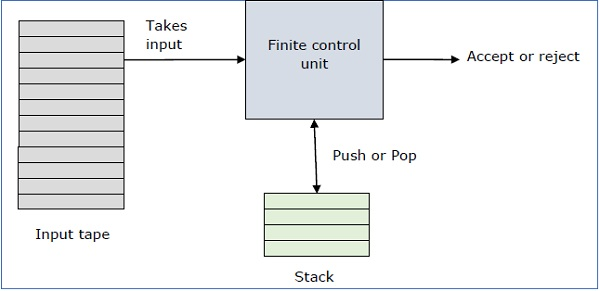A PDA can be formally described as a 7-tuple (Q, ∑, S, δ, q0, I, F) −

• Q is the finite number of states

• is input alphabet

• S is stack symbols

• δ is the transition function: Q × (∑ ∪ {ε}) × S × Q × S*

• q0 is the initial state (q0 ∈ Q)

• I is the initial stack top symbol (I ∈ S)

• F is a set of accepting states (F ∈ Q)

The following diagram shows a transition in a PDA from a state q1 to state q2, labeled as a,b → c −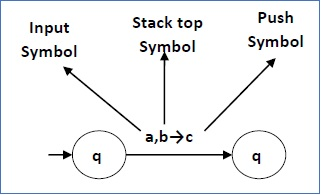This means at state q1, if we encounter an input string ‘a’ and top symbol of the stack is ‘b’, then we pop ‘b’, push ‘c’ on top of the stack and move to state q2.

## Terminologies Related to PDA

### Instantaneous Description

The instantaneous description (ID) of a PDA is represented by a triplet (q, w, s) where

• q is the state

• w is unconsumed input

• s is the stack contents

### Turnstile Notation

The "turnstile" notation is used for connecting pairs of ID's that represent one or many moves of a PDA. The process of transition is denoted by the turnstile symbol "⊢".

Consider a PDA (Q, ∑, S, δ, q0, I, F). A transition can be mathematically represented by the following turnstile notation −

```(p, aw, Tβ) ⊢ (q, w, αb)
```

This implies that while taking a transition from state p to state q, the input symbol ‘a’ is consumed, and the top of the stack ‘T’ is replaced by a new string ‘α’.

Note − If we want zero or more moves of a PDA, we have to use the symbol (⊢*) for it.

# Pushdown Automata Acceptance

There are two different ways to define PDA acceptability.

## Final State Acceptability

In final state acceptability, a PDA accepts a string when, after reading the entire string, the PDA is in a final state. From the starting state, we can make moves that end up in a final state with any stack values. The stack values are irrelevant as long as we end up in a final state.

For a PDA (Q, ∑, S, δ, q0, I, F), the language accepted by the set of final states F is −

L(PDA) = {w | (q0, w, I) ⊢* (q, ε, x), q ∈ F}

for any input stack string x.

## Empty Stack Acceptability

Here a PDA accepts a string when, after reading the entire string, the PDA has emptied its stack.

For a PDA (Q, ∑, S, δ, q0, I, F), the language accepted by the empty stack is −

L(PDA) = {w | (q0, w, I) ⊢* (q, ε, ε), q ∈ Q}

### Example

Construct a PDA that accepts L = {0n 1n | n ≥ 0}

### SolutionThis language accepts L = {ε, 01, 0011, 000111, ............................. }

Here, in this example, the number of ‘a’ and ‘b’ have to be same.

• Initially we put a special symbol ‘\$’ into the empty stack.

• Then at state q2, if we encounter input 0 and top is Null, we push 0 into stack. This may iterate. And if we encounter input 1 and top is 0, we pop this 0.

• Then at state q3, if we encounter input 1 and top is 0, we pop this 0. This may also iterate. And if we encounter input 1 and top is 0, we pop the top element.

• If the special symbol ‘\$’ is encountered at top of the stack, it is popped out and it finally goes to the accepting state q4.

### Example

Construct a PDA that accepts L = { wwR | w = (a+b)* }

Solution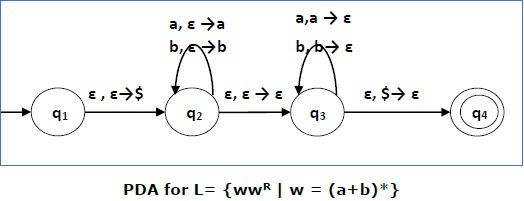Initially we put a special symbol ‘\$’ into the empty stack. At state q2, the w is being read. In state q3, each 0 or 1 is popped when it matches the input. If any other input is given, the PDA will go to a dead state. When we reach that special symbol ‘\$’, we go to the accepting state q4.

# PDA & Context-Free Grammar

If a grammar G is context-free, we can build an equivalent nondeterministic PDA which accepts the language that is produced by the context-free grammar G. A parser can be built for the grammar G.

Also, if P is a pushdown automaton, an equivalent context-free grammar G can be constructed where

L(G) = L(P)

In the next two topics, we will discuss how to convert from PDA to CFG and vice versa.

## Algorithm to find PDA corresponding to a given CFG

Input − A CFG, G = (V, T, P, S)

Output − Equivalent PDA, P = (Q, ∑, S, δ, q0, I, F)

Step 1 − Convert the productions of the CFG into GNF.

Step 2 − The PDA will have only one state {q}.

Step 3 − The start symbol of CFG will be the start symbol in the PDA.

Step 4 − All non-terminals of the CFG will be the stack symbols of the PDA and all the terminals of the CFG will be the input symbols of the PDA.

Step 5 − For each production in the form A → aX where a is terminal and A, X are combination of terminal and non-terminals, make a transition δ (q, a, A).

### Problem

Construct a PDA from the following CFG.

G = ({S, X}, {a, b}, P, S)

where the productions are −

S → XS | ε , A → aXb | Ab | ab

### Solution

Let the equivalent PDA,

P = ({q}, {a, b}, {a, b, X, S}, δ, q, S)

where δ −

δ(q, ε , S) = {(q, XS), (q, ε )}

δ(q, ε , X) = {(q, aXb), (q, Xb), (q, ab)}

δ(q, a, a) = {(q, ε )}

δ(q, 1, 1) = {(q, ε )}

## Algorithm to find CFG corresponding to a given PDA

Input − A CFG, G = (V, T, P, S)

Output − Equivalent PDA, P = (Q, ∑, S, δ, q0, I, F) such that the non- terminals of the grammar G will be {Xwx | w,x ∈ Q} and the start state will be Aq0,F.

Step 1 − For every w, x, y, z ∈ Q, m ∈ S and a, b ∈ ∑, if δ (w, a, ε) contains (y, m) and (z, b, m) contains (x, ε), add the production rule Xwx → a Xyzb in grammar G.

Step 2 − For every w, x, y, z ∈ Q, add the production rule Xwx → XwyXyx in grammar G.

Step 3 − For w ∈ Q, add the production rule Xww → ε in grammar G.

# Pushdown Automata & Parsing

Parsing is used to derive a string using the production rules of a grammar. It is used to check the acceptability of a string. Compiler is used to check whether or not a string is syntactically correct. A parser takes the inputs and builds a parse tree.

A parser can be of two types −

• Top-Down Parser − Top-down parsing starts from the top with the start-symbol and derives a string using a parse tree.

• Bottom-Up Parser − Bottom-up parsing starts from the bottom with the string and comes to the start symbol using a parse tree.

## Design of Top-Down Parser

For top-down parsing, a PDA has the following four types of transitions −

• Pop the non-terminal on the left hand side of the production at the top of the stack and push its right-hand side string.

• If the top symbol of the stack matches with the input symbol being read, pop it.

• Push the start symbol ‘S’ into the stack.

• If the input string is fully read and the stack is empty, go to the final state ‘F’.

### Example

Design a top-down parser for the expression "x+y*z" for the grammar G with the following production rules −

P: S → S+X | X, X → X*Y | Y, Y → (S) | id

Solution

If the PDA is (Q, ∑, S, δ, q0, I, F), then the top-down parsing is −

(x+y*z, I) ⊢(x +y*z, SI) ⊢ (x+y*z, S+XI) ⊢(x+y*z, X+XI)

⊢(x+y*z, Y+X I) ⊢(x+y*z, x+XI) ⊢(+y*z, +XI) ⊢ (y*z, XI)

⊢(y*z, X*YI) ⊢(y*z, y*YI) ⊢(*z,*YI) ⊢(z, YI) ⊢(z, zI) ⊢(ε, I)

## Design of a Bottom-Up Parser

For bottom-up parsing, a PDA has the following four types of transitions −

• Push the current input symbol into the stack.

• Replace the right-hand side of a production at the top of the stack with its left-hand side.

• If the top of the stack element matches with the current input symbol, pop it.

• If the input string is fully read and only if the start symbol ‘S’ remains in the stack, pop it and go to the final state ‘F’.

### Example

Design a top-down parser for the expression "x+y*z" for the grammar G with the following production rules −

P: S → S+X | X, X → X*Y | Y, Y → (S) | id

Solution

If the PDA is (Q, ∑, S, δ, q0, I, F), then the bottom-up parsing is −

(x+y*z, I) ⊢ (+y*z, xI) ⊢ (+y*z, YI) ⊢ (+y*z, XI) ⊢ (+y*z, SI)

⊢(y*z, +SI) ⊢ (*z, y+SI) ⊢ (*z, Y+SI) ⊢ (*z, X+SI) ⊢ (z, *X+SI)

⊢ (ε, z*X+SI) ⊢ (ε, Y*X+SI) ⊢ (ε, X+SI) ⊢ (ε, SI)

# Turing Machine Introduction

A Turing Machine is an accepting device which accepts the languages (recursively enumerable set) generated by type 0 grammars. It was invented in 1936 by Alan Turing.

## Definition

A Turing Machine (TM) is a mathematical model which consists of an infinite length tape divided into cells on which input is given. It consists of a head which reads the input tape. A state register stores the state of the Turing machine. After reading an input symbol, it is replaced with another symbol, its internal state is changed, and it moves from one cell to the right or left. If the TM reaches the final state, the input string is accepted, otherwise rejected.

A TM can be formally described as a 7-tuple (Q, X, ∑, δ, q0, B, F) where −

• Q is a finite set of states

• X is the tape alphabet

• is the input alphabet

• δ is a transition function; δ : Q × X → Q × X × {Left_shift, Right_shift}.

• q0 is the initial state

• B is the blank symbol

• F is the set of final states

### Comparison with the previous automaton

The following table shows a comparison of how a Turing machine differs from Finite Automaton and Pushdown Automaton.

Machine Stack Data Structure Deterministic?
Finite Automaton N.A Yes
Pushdown Automaton Last In First Out(LIFO) No
Turing Machine Infinite tape Yes

### Example of Turing machine

Turing machine M = (Q, X, ∑, δ, q0, B, F) with

• Q = {q0, q1, q2, qf}
• X = {a, b}
• ∑ = {1}
• q0 = {q0}
• B = blank symbol
• F = {qf }

δ is given by −

Tape alphabet symbol Present State ‘q0 Present State ‘q1 Present State ‘q2
a 1Rq1 1Lq0 1Lqf
b 1Lq2 1Rq1 1Rqf

Here the transition 1Rq1 implies that the write symbol is 1, the tape moves right, and the next state is q1. Similarly, the transition 1Lq2 implies that the write symbol is 1, the tape moves left, and the next state is q2.

## Time and Space Complexity of a Turing Machine

For a Turing machine, the time complexity refers to the measure of the number of times the tape moves when the machine is initialized for some input symbols and the space complexity is the number of cells of the tape written.

Time complexity all reasonable functions −

T(n) = O(n log n)

TM's space complexity −

S(n) = O(n)

# Accepted Language & Decided Language

A TM accepts a language if it enters into a final state for any input string w. A language is recursively enumerable (generated by Type-0 grammar) if it is accepted by a Turing machine.

A TM decides a language if it accepts it and enters into a rejecting state for any input not in the language. A language is recursive if it is decided by a Turing machine.

There may be some cases where a TM does not stop. Such TM accepts the language, but it does not decide it.

## Designing a Turing Machine

The basic guidelines of designing a Turing machine have been explained below with the help of a couple of examples.

### Example 1

Design a TM to recognize all strings consisting of an odd number of α’s.

Solution

The Turing machine M can be constructed by the following moves −

• Let q1 be the initial state.

• If M is in q1; on scanning α, it enters the state q2 and writes B (blank).

• If M is in q2; on scanning α, it enters the state q1 and writes B (blank).

• From the above moves, we can see that M enters the state q1 if it scans an even number of α’s, and it enters the state q2 if it scans an odd number of α’s. Hence q2 is the only accepting state.

Hence,

M = {{q1, q2}, {1}, {1, B}, δ, q1, B, {q2}}

where δ is given by −

Tape alphabet symbol Present State ‘q1 Present State ‘q2
α BRq2 BRq1

### Example 2

Design a Turing Machine that reads a string representing a binary number and erases all leading 0’s in the string. However, if the string comprises of only 0’s, it keeps one 0.

Solution

Let us assume that the input string is terminated by a blank symbol, B, at each end of the string.

The Turing Machine, M, can be constructed by the following moves −

• Let q0 be the initial state.

• If M is in q0, on reading 0, it moves right, enters the state q1 and erases 0. On reading 1, it enters the state q2 and moves right.

• If M is in q1, on reading 0, it moves right and erases 0, i.e., it replaces 0’s by B’s. On reaching the leftmost 1, it enters q2 and moves right. If it reaches B, i.e., the string comprises of only 0’s, it moves left and enters the state q3.

• If M is in q2, on reading either 0 or 1, it moves right. On reaching B, it moves left and enters the state q4. This validates that the string comprises only of 0’s and 1’s.

• If M is in q3, it replaces B by 0, moves left and reaches the final state qf.

• If M is in q4, on reading either 0 or 1, it moves left. On reaching the beginning of the string, i.e., when it reads B, it reaches the final state qf.

Hence,

M = {{q0, q1, q2, q3, q4, qf}, {0,1, B}, {1, B}, δ, q0, B, {qf}}

where δ is given by −

Tape alphabet symbol Present State ‘q0 Present State ‘q1 Present State ‘q2 Present State ‘q3 Present State ‘q4
0 BRq1 BRq1 ORq2 - OLq4
1 1Rq2 1Rq2 1Rq2 - 1Lq4
B BRq1 BLq3 BLq4 OLqf BRqf

# Multi-tape Turing Machine

Multi-tape Turing Machines have multiple tapes where each tape is accessed with a separate head. Each head can move independently of the other heads. Initially the input is on tape 1 and others are blank. At first, the first tape is occupied by the input and the other tapes are kept blank. Next, the machine reads consecutive symbols under its heads and the TM prints a symbol on each tape and moves its heads.A Multi-tape Turing machine can be formally described as a 6-tuple (Q, X, B, δ, q0, F) where −

• Q is a finite set of states

• X is the tape alphabet

• B is the blank symbol

• δ is a relation on states and symbols where

δ: Q × Xk → Q × (X × {Left_shift, Right_shift, No_shift })k

where there is k number of tapes

• q0 is the initial state

• F is the set of final states

Note − Every Multi-tape Turing machine has an equivalent single-tape Turing machine.

# Multi-track Turing Machine

Multi-track Turing machines, a specific type of Multi-tape Turing machine, contain multiple tracks but just one tape head reads and writes on all tracks. Here, a single tape head reads n symbols from n tracks at one step. It accepts recursively enumerable languages like a normal single-track single-tape Turing Machine accepts.

A Multi-track Turing machine can be formally described as a 6-tuple (Q, X, ∑, δ, q0, F) where −

• Q is a finite set of states

• X is the tape alphabet

• is the input alphabet

• δ is a relation on states and symbols where

δ(Qi, [a1, a2, a3,....]) = (Qj, [b1, b2, b3,....], Left_shift or Right_shift)

• q0 is the initial state

• F is the set of final states

Note − For every single-track Turing Machine S, there is an equivalent multi-track Turing Machine M such that L(S) = L(M).

# Non-Deterministic Turing Machine

In a Non-Deterministic Turing Machine, for every state and symbol, there are a group of actions the TM can have. So, here the transitions are not deterministic. The computation of a non-deterministic Turing Machine is a tree of configurations that can be reached from the start configuration.

An input is accepted if there is at least one node of the tree which is an accept configuration, otherwise it is not accepted. If all branches of the computational tree halt on all inputs, the non-deterministic Turing Machine is called a Decider and if for some input, all branches are rejected, the input is also rejected.

A non-deterministic Turing machine can be formally defined as a 6-tuple (Q, X, ∑, δ, q0, B, F) where −

• Q is a finite set of states

• X is the tape alphabet

• is the input alphabet

• δ is a transition function;

δ : Q × X → P(Q × X × {Left_shift, Right_shift}).

• q0 is the initial state

• B is the blank symbol

• F is the set of final states

# Semi-Infinite Tape Turing Machine

A Turing Machine with a semi-infinite tape has a left end but no right end. The left end is limited with an end marker.It is a two-track tape −

• Upper track − It represents the cells to the right of the initial head position.

• Lower track − It represents the cells to the left of the initial head position in reverse order.

The infinite length input string is initially written on the tape in contiguous tape cells.

The machine starts from the initial state q0 and the head scans from the left end marker ‘End’. In each step, it reads the symbol on the tape under its head. It writes a new symbol on that tape cell and then it moves the head either into left or right one tape cell. A transition function determines the actions to be taken.

It has two special states called accept state and reject state. If at any point of time it enters into the accepted state, the input is accepted and if it enters into the reject state, the input is rejected by the TM. In some cases, it continues to run infinitely without being accepted or rejected for some certain input symbols.

Note − Turing machines with semi-infinite tape are equivalent to standard Turing machines.

# Linear Bounded Automata

A linear bounded automaton is a multi-track non-deterministic Turing machine with a tape of some bounded finite length.

Length = function (Length of the initial input string, constant c)

Here,

Memory information ≤ c × Input information

The computation is restricted to the constant bounded area. The input alphabet contains two special symbols which serve as left end markers and right end markers which mean the transitions neither move to the left of the left end marker nor to the right of the right end marker of the tape.

A linear bounded automaton can be defined as an 8-tuple (Q, X, ∑, q0, ML, MR, δ, F) where −

• Q is a finite set of states

• X is the tape alphabet

• is the input alphabet

• q0 is the initial state

• ML is the left end marker

• MR is the right end marker where MR ≠ ML

• δ is a transition function which maps each pair (state, tape symbol) to (state, tape symbol, Constant ‘c’) where c can be 0 or +1 or -1

• F is the set of final states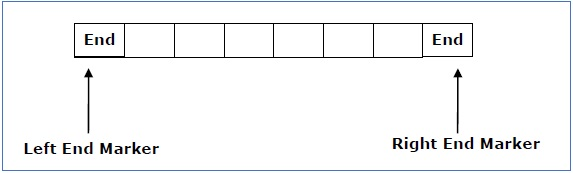A deterministic linear bounded automaton is always context-sensitive and the linear bounded automaton with empty language is undecidable..

# Language Decidability

A language is called Decidable or Recursive if there is a Turing machine which accepts and halts on every input string w. Every decidable language is Turing-Acceptable.A decision problem P is decidable if the language L of all yes instances to P is decidable.

For a decidable language, for each input string, the TM halts either at the accept or the reject state as depicted in the following diagram −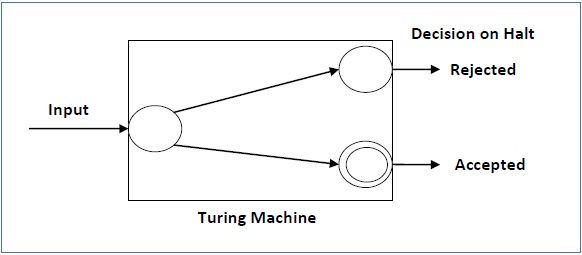## Example 1

Find out whether the following problem is decidable or not −

Is a number ‘m’ prime?

### Solution

Prime numbers = {2, 3, 5, 7, 11, 13, …………..}

Divide the number ‘m’ by all the numbers between ‘2’ and ‘√m’ starting from ‘2’.

If any of these numbers produce a remainder zero, then it goes to the “Rejected state”, otherwise it goes to the “Accepted state”. So, here the answer could be made by ‘Yes’ or ‘No’.

Hence, it is a decidable problem.

## Example 2

Given a regular language L and string w, how can we check if w ∈ L?

### Solution

Take the DFA that accepts L and check if w is accepted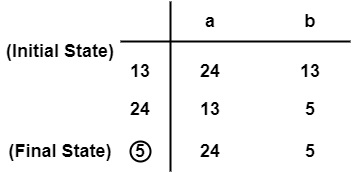Some more decidable problems are −

• Does DFA accept the empty language?
• Is L1 ∩ L2 = ∅ for regular sets?

Note

• If a language L is decidable, then its complement L' is also decidable

• If a language is decidable, then there is an enumerator for it.

# Undecidable Languages

For an undecidable language, there is no Turing Machine which accepts the language and makes a decision for every input string w (TM can make decision for some input string though). A decision problem P is called “undecidable” if the language L of all yes instances to P is not decidable. Undecidable languages are not recursive languages, but sometimes, they may be recursively enumerable languages.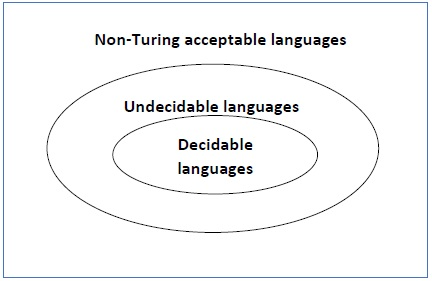## Example

• The halting problem of Turing machine
• The mortality problem
• The mortal matrix problem
• The Post correspondence problem, etc.

# Turing Machine Halting Problem

Input − A Turing machine and an input string w.

Problem − Does the Turing machine finish computing of the string w in a finite number of steps? The answer must be either yes or no.

Proof − At first, we will assume that such a Turing machine exists to solve this problem and then we will show it is contradicting itself. We will call this Turing machine as a Halting machine that produces a ‘yes’ or ‘no’ in a finite amount of time. If the halting machine finishes in a finite amount of time, the output comes as ‘yes’, otherwise as ‘no’. The following is the block diagram of a Halting machine −Now we will design an inverted halting machine (HM)’ as −

• If H returns YES, then loop forever.

• If H returns NO, then halt.

The following is the block diagram of an ‘Inverted halting machine’ −Further, a machine (HM)2 which input itself is constructed as follows −

• If (HM)2 halts on input, loop forever.
• Else, halt.

Here, we have got a contradiction. Hence, the halting problem is undecidable.

# Rice Theorem

Rice theorem states that any non-trivial semantic property of a language which is recognized by a Turing machine is undecidable. A property, P, is the language of all Turing machines that satisfy that property.

## Formal Definition

If P is a non-trivial property, and the language holding the property, Lp , is recognized by Turing machine M, then Lp = {<M> | L(M) ∈ P} is undecidable.

## Description and Properties

• Property of languages, P, is simply a set of languages. If any language belongs to P (L ∈ P), it is said that L satisfies the property P.

• A property is called to be trivial if either it is not satisfied by any recursively enumerable languages, or if it is satisfied by all recursively enumerable languages.

• A non-trivial property is satisfied by some recursively enumerable languages and are not satisfied by others. Formally speaking, in a non-trivial property, where L ∈ P, both the following properties hold:

• Property 1 − There exists Turing Machines, M1 and M2 that recognize the same language, i.e. either ( <M1>, <M2> ∈ L ) or ( <M1>,<M2> ∉ L )

• Property 2 − There exists Turing Machines M1 and M2, where M1 recognizes the language while M2 does not, i.e. <M1> ∈ L and <M2> ∉ L

## Proof

Suppose, a property P is non-trivial and φ ∈ P.

Since, P is non-trivial, at least one language satisfies P, i.e., L(M0) ∈ P , ∋ Turing Machine M0.

Let, w be an input in a particular instant and N is a Turing Machine which follows −

On input x

• Run M on w
• If M does not accept (or doesn't halt), then do not accept x (or do not halt)
• If M accepts w then run M0 on x. If M0 accepts x, then accept x.

A function that maps an instance ATM = {<M,w>| M accepts input w} to a N such that

• If M accepts w and N accepts the same language as M0, Then L(M) = L(M0) ∈ p
• If M does not accept w and N accepts φ, Then L(N) = φ ∉ p

Since ATM is undecidable and it can be reduced to Lp, Lp is also undecidable.

# Post Correspondence Problem

The Post Correspondence Problem (PCP), introduced by Emil Post in 1946, is an undecidable decision problem. The PCP problem over an alphabet ∑ is stated as follows −

Given the following two lists, M and N of non-empty strings over ∑ −

M = (x1, x2, x3,………, xn)

N = (y1, y2, y3,………, yn)

We can say that there is a Post Correspondence Solution, if for some i1,i2,………… ik, where 1 ≤ ij ≤ n, the condition xi1 …….xik = yi1 …….yik satisfies.

## Example 1

Find whether the lists

M = (abb, aa, aaa) and N = (bba, aaa, aa)

have a Post Correspondence Solution?

### Solution

x1 x2 x3
M Abb aa aaa
N Bba aaa aa

Here,

x2x1x3 = ‘aaabbaaa’

and y2y1y3 = ‘aaabbaaa’

We can see that

x2x1x3 = y2y1y3

Hence, the solution is i = 2, j = 1, and k = 3.

## Example 2

Find whether the lists M = (ab, bab, bbaaa) and N = (a, ba, bab) have a Post Correspondence Solution?

### Solution

x1 x2 x3
M ab bab bbaaa
N a ba bab

In this case, there is no solution because −

| x2x1x3 | ≠ | y2y1y3 | (Lengths are not same)

Hence, it can be said that this Post Correspondence Problem is undecidable.